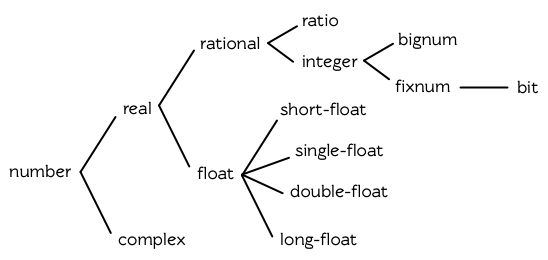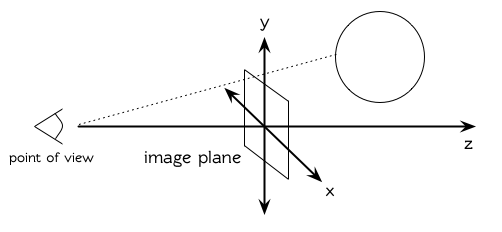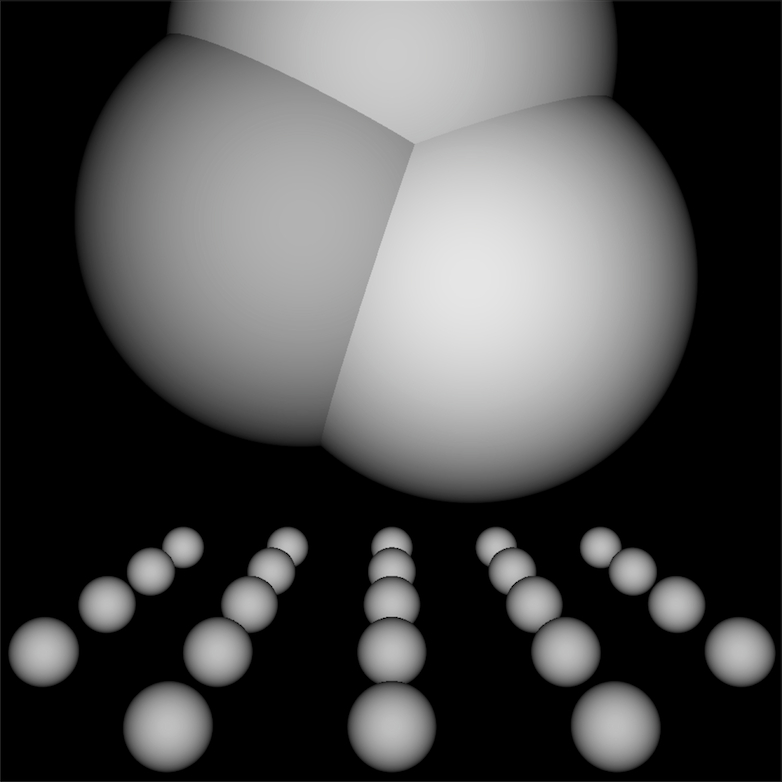# 第九章：數字¶

## 9.1 型別 (Types)¶

Common Lisp 提供了四種不同型別的數字：整數、浮點數、比值與複數。本章所講述的函數適用於所有型別的數字。有幾個不能用在複數的函數會特別說明。1. 如果數值函數接受一個或多個浮點數作爲參數，則返回值會是浮點數 (或是由浮點陣列成的複數)。所以 (+ 1.0 2) 求值爲 3.0 ，而 (+ #c(0 1.0) 2) 求值爲 #c(2.0 1.0)
2. 可約分的比值會被轉換成最簡分數。所以 (/ 10 2) 會返回 5
3. 若計算過程中複數的虛部變成 0 時，則複數會被轉成實數 。所以 (+ #c(1 -1) #c(2 1)) 求值成 3

> (list (ratiop 2/2) (complexp #c(1 0)))
(NIL NIL)


## 9.2 轉換及取出 (Conversion and Extraction)¶

Lisp 提供四種不同型別的數字的轉換及取出位數的函數。函數 float 將任何實數轉換成浮點數:

> (mapcar #'float '(1 2/3 .5))
(1.0 0.6666667 0.5)


> (truncate 1.3)
1
0.29999995


(defun palindrome? (x)
(let ((mid (/ (length x) 2)))
(equal (subseq x 0 (floor mid))
(reverse (subseq x (ceiling mid))))))


truncate 一樣， floorceiling 也返回傳入參數與第一個返回值的差，作爲第二個返回值。

> (floor 1.5)
1
0.5


(defun our-truncate (n)
(if (> n 0)
(floor n)
(ceiling n)))


> (mapcar #'round '(-2.5 -1.5 1.5 2.5))
(-2 -2 2 2)


> (mapcar #'signum '(-2 -0.0 0.0 0 .5 3))
(-1 -0.0 0.0 0 1.0 1)


## 9.3 比較 (Comparison)¶

> (= 1 1.0)
T
> (eql 1 1.0)
NIL


= 比起 eql 來得寬鬆，但參數的型別需一致。

(<= w x y z)


(and (<= w x) (<= x y) (<= y z))


(/= w x y z)


(and (/= w x) (/= w y) (/= w z)
(/= x y) (/= y z) (/= y z))


> (list (minusp -0.0) (zerop -0.0))
(NIL T)


> (list (max 1 2 3 4 5) (min 1 2 3 4 5))
(5 1)


## 9.4 算術 (Arithematic)¶

(- x y z)


(- (- x y) z)


incfdecf 分別遞增與遞減數字。這樣形式的表達式 (incf x n) 類似於 (setf x (+ x n)) 的效果，而 (decf x n) 類似於 (setf x (- x n)) 的效果。這兩個形式裡，第二個參數皆是選擇性給入的，預設值爲 1

> (/ 3)
1/3


(/ x y z)


(/ (/ x y) z)


> (/ 365 12)
365/12


> (float 365/12)
30.416666


## 9.5 指數 (Exponentiation)¶

> (expt 2 5)
32


> (log 32 2)
5.0


> (exp 2)
7.389056


> (log 7.389056)
2.0


> (expt 27 1/3)
3.0


> (sqrt 4)
2.0


## 9.6 三角函數 (Trigometric Functions)¶

> (let ((x (/ pi 4)))
(list (sin x) (cos x) (tan x)))
(0.7071067811865475d0 0.7071067811865476d0 1.0d0)
;;; 譯註: CCL 1.8  SBCL 1.0.55 下的結果是
;;; (0.7071067811865475D0 0.7071067811865476D0 0.9999999999999999D0)


## 9.7 表示法 (Representations)¶

Common Lisp 沒有限制整數的大小。可以塞進一個字（word）記憶體的小整數稱爲定長數(fixnums)。在計算過程中，整數無法塞入一個字時，Lisp 切換至使用多個字的表示法（一個大數 「bignum」）。所以整數的大小限製取決於實體記憶體，而不是語言。

> (values most-positive-fixnum most-negative-fixnum)
536870911
-536870912
;;; 譯註: CCL 1.8 的結果爲
1152921504606846975
-1152921504606846976
;;; SBCL 1.0.55 的結果爲
4611686018427387903
-4611686018427387904


> (typep 1 'fixnum)
T
> (type (1+ most-positive-fixnum) 'bignum)
T


（譯註: s 爲短浮點、 f 爲單浮點、 d 爲雙浮點、 l 爲長浮點。）

> (* most-positive-long-float 10)
Error: floating-point-overflow


## 9.8 範例：追蹤光線 (Example: Ray-Tracing)¶

(defun sq (x) (* x x))

(defun mag (x y z)
(sqrt (+ (sq x) (sq y) (sq z))))

(defun unit-vector (x y z)
(let ((d (mag x y z)))
(values (/ x d) (/ y d) (/ z d))))

(defstruct (point (:conc-name nil))
x y z)

(defun distance (p1 p2)
(mag (- (x p1) (x p2))
(- (y p1) (y p2))
(- (z p1) (z p2))))

(defun minroot (a b c)
(if (zerop a)
(/ (- c) b)
(let ((disc (- (sq b) (* 4 a c))))
(unless (minusp disc)
(let ((discrt (sqrt disc)))
(min (/ (+ (- b) discrt) (* 2 a))
(/ (- (- b) discrt) (* 2 a))))))))


> (multiple-value-call #'mag (unit-vector 23 12 47))
1.0


$x = \dfrac{-b \pm \sqrt{b^2 - 4ac}}{2a}$

surface 結構用來表示模擬世界中的物體。更精確的說，它會被 included 至定義具體型別物體的結構裡，像是球體 (spheres)。 surface 結構本身只包含一個欄位: 一個 color 範圍從 0 (黑色) 至 1 (白色)。

(defstruct surface color)

(defparameter *world* nil)
(defconstant eye (make-point :x 0 :y 0 :z 200))

(defun tracer (pathname &optional (res 1))
(with-open-file (p pathname :direction :output)
(format p "P2 ~A ~A 255" (* res 100) (* res 100))
(let ((inc (/ res)))
(do ((y -50 (+ y inc)))
((< (- 50 y) inc))
(do ((x -50 (+ x inc)))
((< (- 50 x) inc))
(print (color-at x y) p))))))

(defun color-at (x y)
(multiple-value-bind (xr yr zr)
(unit-vector (- x (x eye))
(- y (y eye))
(- 0 (z eye)))
(round (* (sendray eye xr yr zr) 255))))

(defun sendray (pt xr yr zr)
(multiple-value-bind (s int) (first-hit pt xr yr zr)
(if s
(* (lambert s int xr yr zr) (surface-color s))
0)))

(defun first-hit (pt xr yr zr)
(let (surface hit dist)
(dolist (s *world*)
(let ((h (intersect s pt xr yr zr)))
(when h
(let ((d (distance h pt)))
(when (or (null dist) (< d dist))
(setf surface s hit h dist d))))))
(values surface hit)))

(defun lambert (s int xr yr zr)
(multiple-value-bind (xn yn zn) (normal s int)
(max 0 (+ (* xr xn) (* yr yn) (* zr zn)))))$i = N·L$

sendray 這個值會乘上平面的顏色 (即便是有好的照明，一個暗的平面還是暗的)來決定該點之後總體亮度。

(defstruct (sphere (:include surface))

(defun defsphere (x y z r c)
(let ((s (make-sphere
:center (make-point :x x :y y :z z)
:color  c)))
(push s *world*)
s))

(defun intersect (s pt xr yr zr)
(funcall (typecase s (sphere #'sphere-intersect))
s pt xr yr zr))

(defun sphere-intersect (s pt xr yr zr)
(let* ((c (sphere-center s))
(n (minroot (+ (sq xr) (sq yr) (sq zr))
(* 2 (+ (* (- (x pt) (x c)) xr)
(* (- (y pt) (y c)) yr)
(* (- (z pt) (z c)) zr)))
(+ (sq (- (x pt) (x c)))
(sq (- (y pt) (y c)))
(sq (- (z pt) (z c)))
(if n
(make-point :x  (+ (x pt) (* n xr))
:y  (+ (y pt) (* n yr))
:z  (+ (z pt) (* n zr))))))

(defun normal (s pt)
(funcall (typecase s (sphere #'sphere-normal))
s pt))

(defun sphere-normal (s pt)
(let ((c (sphere-center s)))
(unit-vector (- (x c) (x pt))
(- (y c) (y pt))
(- (z c) (z pt)))))


$r = \sqrt{ (x_0 + nx_r - x_c)^2 + (y_0 + ny_r - y_c)^2 + (z_0 + nz_r - z_c)^2 }$

$an^2 + bn + c = 0$

$\begin{split}a = x_r^2 + y_r^2 + z_r^2\\b = 2((x_0-x_c)x_r + (y_0-y_c)y_r + (z_0-z_c)z_r)\\c = (x_0-x_c)^2 + (y_0-y_c)^2 + (z_0-z_c)^2 - r^2\end{split}$

(譯註：PGM 可移植灰度圖格式，更多資訊參見 wiki )

(defun ray-test (&optional (res 1))
(setf *world* nil)
(defsphere 0 -300 -1200 200 .8)
(defsphere -80 -150 -1200 200 .7)
(defsphere 70 -100 -1200 200 .9)
(do ((x -2 (1+ x)))
((> x 2))
(do ((z 2 (1+ z)))
((> z 7))
(defsphere (* x 200) 300 (* z -400) 40 .75)))
(tracer (make-pathname :name "spheres.pgm") res))## Chapter 9 總結 (Summary)¶

1. Common Lisp 提供整數 (integers)、比值 (ratios)、浮點數 (floating-point numbers)以及複數 (complex numbers)。
2. 數字可以被約分或轉換 (converted)，而它們的位數 (components)可以被取出。
3. 用來比較數字的謂詞可以接受任意數量的參數，以及比較下一數對 (successive pairs) ── /= 函數除外，它是用來比較所有的數對 (pairs)。
4. Common Lisp 幾乎提供你在低階科學計算機可以看到的數值函數。同樣的函數普遍可應用在多種型別的數字上。
5. Fixnum 是小至可以塞入一個字 (word)的整數。它們在必要時會悄悄但花費昂貴地轉成大數 (bignum)。Common Lisp 提供最多四種浮點數。每一個浮點表示法的限制是實現相關的 (implementation-dependent)常數。
6. 一個光線追蹤器 (ray-tracer)通過追蹤光線來產生圖像，使得每一像素回到模擬的世界。

## Chapter 9 練習 (Exercises)¶

1. 定義一個函數，接受一個實數列表，若且唯若 (iff)它們是非遞減 (nondecreasing)順序時返回真。
2. 定義一個函數，接受一個整數 cents 並返回四個值，將數字用 25- , 10- , 5- , 1- 來顯示，使用最少數量的硬幣。(譯註: 25- 是 25 美分，以此類推)
3. 一個遙遠的星球住著兩種生物， wigglies 與 wobblies 。 Wigglies 與 wobblies 唱歌一樣厲害。每年都有一個比賽來選出十大最佳歌手。下面是過去十年的結果:
YEAR 1 2 3 4 5 6 7 8 9 10
WIGGLIES 6 5 6 4 5 5 4 5 6 5
WOBBLIES 4 5 4 6 5 5 6 5 4 5

1. 定義一個函數，接受 8 個表示二維空間中兩個線段端點的實數，若線段沒有相交，則返回假，或返回兩個值表示相交點的 x 座標與 y 座標。
2. 假設 f 是一個接受一個 (實數) 參數的函數，而 minmax 是有著不同正負號的非零實數，使得 f 對於參數 i 有一個根 (返回零)並滿足 min < i < max 。定義一個函數，接受四個參數， f , min , max 以及 epsilon ，並返回一個 i 的近似值，準確至正負 epsilon 之內。
3. Honer’s method 是一個有效率求出多項式的技巧。要找到 $$ax^3+bx^2+cx+d$$ 你對 x(x(ax+b)+c)+d 求值。定義一個函數，接受一個或多個參數 ── x 的值伴隨著 n 個實數，用來表示 (n-1) 次方的多項式的係數 ── 並用 Honer’s method 計算出多項式的值。
1. 你的 Common Lisp 實現使用了幾個位元來表示定長數？
2. 你的 Common Lisp 實現提供幾種不同的浮點數？

  當 format 取整顯示時，它不保證會取成偶數或奇數。見 125 頁 (譯註: 7.4 節)。